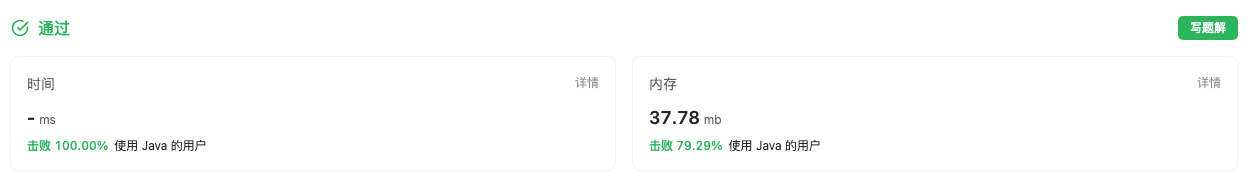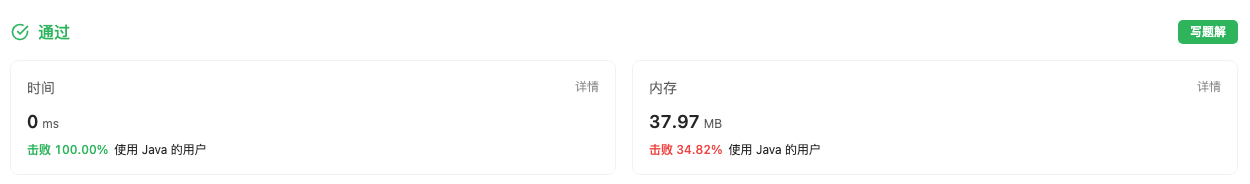#### 198. 打家劫舍

###### 来源: 每日一题 2023.08.18 延展

``````输入：[1,2,3,1]

偷窃到的最高金额 = 1 + 3 = 4 。
``````

``````输入：[2,7,9,3,1]

偷窃到的最高金额 = 2 + 9 + 1 = 12 。
``````

• `1 <= nums.length <= 100`
• `0 <= nums[i] <= 400`
``````class Solution {
public int rob(int[] nums) {

}
}
``````

#### 分析与题解

• 动态规划

这个题目是典型的动态规划问题, 我们需要考虑的条件是小偷不通同时进入两个相邻的房间, 所以如果想要在 `下标i` 点偷的钱最多, 那么就需要有以下两种情况.

• 情况1: 当前房屋不偷, 但是前一个房屋偷

• 情况2: 前一个房屋不偷, 但是当前房屋偷.

那么就会有如下的状态转移方程.

``````dp[i] = Math.max(dp[i - 2] + nums[i], dp[i -1]);
``````

对于初始状态, 我们需要设定 `i = 0``i = 1` 两种情况.

``````dp = nums;
dp = Math.max(nums, nums);
``````

当然了, 我们还需要判断只有一个元素的边界条件.

``````if (nums.length == 1) {
return nums;
}
``````

整体的解题思路如下所示.

``````class Solution {
public int rob(int[] nums) {
if (nums.length == 1) {
return nums;
}
// 只有两种初始情况
int[] dp = new int[nums.length];
dp = nums;
dp = Math.max(nums, nums);
for(int i = 2; i < nums.length; i++) {
dp[i] = Math.max(dp[i - 2] + nums[i], dp[i -1]);
}
return dp[nums.length - 1];
}
}
``````

复杂度分析:

• 时间复杂度: O(n), 时间复杂度与`nums`长度相关.
• 空间复杂度: O(n), `dp` 长度与 `nums` 长度成线性比例关系.

结果如下所示.• 动态规划(优化)

上面我们使用了动态规划, 但是实际上我们只需要关注 dp[i-1] 和 dp[i-2] 两个值即可, 所以我们可以利用常量来解决这个问题, 而并不需要使用 `dp数组`.

``````class Solution {
public int rob(int[] nums) {
if (nums.length == 1) {
return nums;
}
int firstMax = nums;
int secondMax = Math.max(firstMax, nums);
int result = secondMax;
int temp = secondMax;
for(int i = 2; i < nums.length; i++) {
result = Math.max(result, Math.max(firstMax + nums[i], secondMax));
temp = secondMax;
secondMax = Math.max(firstMax + nums[i], secondMax);
firstMax = temp;
}
return result;
}
}
``````

复杂度分析:

• 时间复杂度: O(n), 时间复杂度与`nums`长度相关.
• 空间复杂度: O(1), 常量级别的空间复杂度.

结果如下所示.IT界无底坑洞栋主 欢迎加Q骚扰:676758285